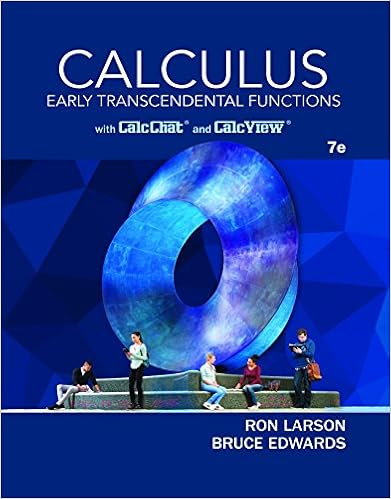# 5 12 points using the fundamental theorem of calculus

• Notes
• 6

This preview shows page 3 - 6 out of 6 pages.

##### We have textbook solutions for you!
The document you are viewing contains questions related to this textbook.The document you are viewing contains questions related to this textbook.
Chapter 7 / Exercise 25
Calculus: Early Transcendental Functions
Edwards/LarsonExpert Verified
5. (12 points) Using the Fundamental Theorem of Calculus, compute dF dx in each case below. a. F ( x ) = R x 4 sin( t ) dt
b. F ( x ) = R 0 e x cos(2 t ) dt
##### We have textbook solutions for you!
The document you are viewing contains questions related to this textbook.The document you are viewing contains questions related to this textbook.
Chapter 7 / Exercise 25
Calculus: Early Transcendental Functions
Edwards/LarsonExpert Verified
Name: ID: Section: 4 6. (07 points) Let g ( x ) = R x 0 f ( t ) dt , where f is the fuction whose graph is drawn below. Using the figure and your knowledge of calculus, fill in the table. You need not justify your answers for this problem. g (0) = g 0 (1) = f (0) = g (2) = g (3) - g (0) = g 0 (4) = f (3) - f (0) =
Name: ID: Section: 5 7. (12 points) Consider the definite integral Z 3 0 ( x 2 + 1) dx. a. Write down the partition of the interval [0 , 3] into n equal sub-intervals. [You may do this by listing the endpoints of the intervals with · · · after the pattern is established, but it is most helpful to write the general form of the k th endpoint. Equiv- alently you can specify the k th interval as [ a k , a k +1 ] giving the values for a k explicitly, in terms of k .]
b. Write down the Riemann Sum for the definite integral above, using the partition from part (a) and the rightmost endpoint of each interval as your c k (evaluation point). [The sum should be as specific as possible, not a general looking Riemann Sum with f ’s and c k ’s and Δ’s symbols. Instead it should contain k ’s and n ’s and numbers, and sigma notation . But beyond that, you need not simplify further.]
•••